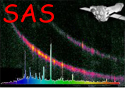XMM-Newton Science Analysis System

arfgen (arfgen-1.104) [xmmsas_20230412_1735-21.0.0]

## Example 4: Specifying an external detector map for an extended source

Here is an example illustrating how to pass an externally-created detector map (section 5.3.2) to the task:

arfgen spectrumset=spectrum.pha detmaptype=dataset detmaparray=detmap.ds
extendedsource=yes

Generates a dataset ancillaryfile.ds, weighting the arf spatially using the first array found in the dataset detmap.ds. The effective area, filter transmission and quantum efficiency curve are included in the ARF. An ARF for an extended source is generated.

In principle any image covering the celestial coordinates of the spectrum extraction region may be used. Typically an XMM image in either detector or sky (X/Y) coordinates generated by evselect or xmmselect would be used. e.g. to create a detector coordinate image covering the regionandof size 20x20 pixels use the command:

evselect table='0001_0000010010_M1S00100IME.FIT:EVENTS'
destruct=false withfilteredset=true
withimageset=true imageset=detmap.ds xcolumn=DETX ycolumn=DETY
withxranges=true ximagemin=-1500 ximagemax=1500 withyranges=true
yimagemin=-1500 yimagemax=1500 imagebinning='imageSize'
ximagesize=20 yimagesize=20
writedss=true updateexposure=true

To use this image to also correct for flux lost to bad pixels and chip gaps use:

arfgen spectrumset=spectrum.pha detmaptype=dataset detmaparray=detmap.ds